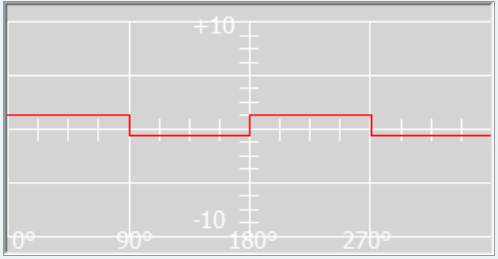# Understanding Wave Cycle Multiplier.

1. Consider the square wave; when X = 1 the output waveform window shows a single transition; when you set it to X = 2 you then see two transitions (or subwaves).When starting the generator this output waveform is sent to the XM generator as an 'arbitrary waveform'.

The XM generator uses this waveform, and if it 'plays' the waveform at 100 Hz, it will actually be outputing 200 Hz, because each one consists of two transitions.

2. What is being considered here is the maximum frequency (capacity) that the generator can send out, which is 5MHz. Now, take for example, your need to use 10MHz. This exceeds generator's capacity, so to avoid overworking the generator, you will need to divide the 10MHz by 2 to send maximum of 5MHz at a time in half a second, then the other 5MHz is also sent in half a second. In one second you would have sent 10MHz. If you look at the waveform, you will notice it became two sub-waves. The generator will only show the 5MHz, so to double check your generator's accurate transmission, you will multiply the generator's LED display of 5MHz by the value of X which is 2. So 5MHz x 2=10MHz.

http://www.spooky2.com/forums/viewtopic.php?f=5&t=6157

1 out of 1 found this helpful